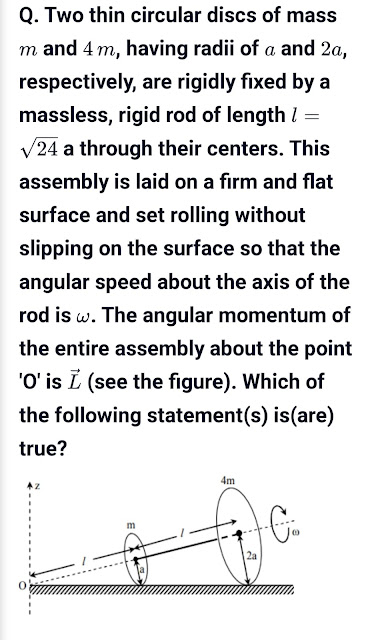## 8 Apr 2021

### For a dealer A, the list price of an article is Rs. 27000, which he sells to dealer B at some lower price. Further, dealer B sells the same article to a customer at its list price. If the rate of GST is 8% and dealer B paid a tax, under GST, equal to Rs. 1944 to the government, find the amount (inclusive of GST) paid by dealer B

sorry on 7th line its 8/100 x 27000

1.Why is it 27000-x?

1.Because x lower price so price of the article is 27000-x

2.The answer is 26244. It is given in my book

1.I think the answer is wrong

3.Output GST = 8/100 x 27000

4.1.Yes in book answer is 26244 if we calculate 24300+1944 then answer will come 26244, but here X which is equal to 23400 is some price which reduce, so cost price for B is 27000-24300=2700

5.Jay kumar pandit27 May 2021 at 01:11

You are right, thanks for this information my school teacher says answer given in the book is correct now I can clarify my teacher 26244 is not the correct answer.

6.Thanks sir

7.Can you please tell in which book this question is given....plz plz

1.in selina publication book

8.Ist of all this question has a misprint. Dealer B will purchase the article at ₹2700+tax on it according to question. Which is practically incorrect as he purchased at rs 2700 and sell at rs 27000

1.Though the question is misprint, we have to solve the question and its also possible that CP is 2700 and Sp is 27000 .